# What is meant by A phase and components?

## The Phase Rule statement

– The phase Rule is an important generalization dealing with the behaviour of heterogeneous systems.

– In general it may be said that with the application of phase rule it is possible to predict qualitatively by means of a diagram the effect of changing pressure, temperature and concentration on a heterogeneous system in equilibrium.

– This relationship governing all heterogeneous equilibria was first discovered as early as 1874 by an American physicist Willard Gibbs.

– Gibb’s Phase Rule is free from flaws and limitations which are a common feature of all other generalizations of Physical Chemistry based on hypothetical assumptions as to the nature of the constitution of matter. It may be stated mathematically as follows :

F = C – P + 2

– where:

F = the number of degrees of freedom,

C = the number of components

P = the number of phases of the system.

– The terms(phase), (component), and (degree of freedom) involved in the statement of the Phase Rule have a special significance and a clear understanding of these terms is essential before we proceed further with the subject.

## What is meant by A phase?

A phase may be defined as:

any homogeneous part of a system having all physical and chemical properties the same throughout.

– A system may consist of one phase or more than one phases.

(1) A system containing only liquid water is one-phase or 1-phase system (P = 1)

(2) A system containing liquid water and water vapour (a gas) is a two-phase or 2-phase system (P = 2).

(3) A system containing liquid water, water vapour and solid ice is a three-phase or 3-phase system.

• A system consisting of one phase only is called a homogeneous system.
• A system consisting of two or more phases is called a heterogeneous system.

## Explanation of the Term (Phase)

– Ordinarily three states of matter-gas, liquid, and solid are known as phases.

– However in phase rule, a uniform part of a system in equilibrium is termed a (phase).

– Thus a liquid or a solid mixture could have two or more phases.

– Let us consider a few examples to understand the meaning of the term phase as encountered in
phase rule.

### (1) Pure substances

– A pure substance (solid, liquid, or gas) made of one chemical species only, is considered as one phase.

– Thus oxygen (O2), benzene (C6H6), and ice (H2O) are all 1-phase systems.

– It must be remembered that a phase may or may not be continuous.

– Thus, whether ice is present in one block or many pieces, it is considered one phase.

### (2) Mixtures of gases.

– All gases mix freely to form homogeneous mixtures.

– Therefore any mixture of gases, say O2 and N2, is a 1-phase system.

### (3) Miscible liquids.

– Two completely miscible liquids yield a uniform solution.

– Thus a solution of ethanol and water is a 1-phase system.

### (4) Non-miscible liquids.

– A mixture of two non-miscible liquids on standing forms two separate layers.

– Hence a mixture of chloroform (CHCl3) and water constitutes a 2-phase system.

### (5) Aqueous solutions.

– An aqueous solution of a solid substance such as sodium chloride (or sugar) is uniform throughout.

– Therefore it is a 1-phase system. However, a saturated solution of sodium chloride in contact with excess solid sodium chloride is a 2-phase system.

### (6) Mixtures of solids.

(i) By definition, a phase must have throughout the same physical and chemical properties.

Ordinary sulphur as it occurs in nature is a mixture of monoclinic and rhombic sulphur.

– These allotropes of sulphur consist of the same chemical species but differ in physical properties. Thus mixture of two allotropes is a 2-phase system.

(ii) A mixture of two or more chemical substances contains as many phases.

– Each of these substances having different physical and chemical properties makes a separate phase.

– Thus a mixture of calcium carbonate (CaCO3) and calcium oxide (CaO) constitutes two phases.

– Let us consider the equilibrium system : the Decomposition of Calcium carbonate.

– When calcium carbonate is heated in a closed vessel, we have: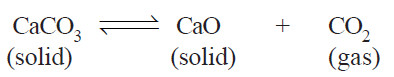There are two solid phases and one gas phase. Hence it is a 3-phase system.

## What is meant by A components ?

– A system ‘C’ in the Phase Rule equation stands for the number of components of a system in equilibrium.

– The term component may be defined as :

the least number of independent chemical constituents in terms of which the composition of every phase can be expressed by means of a chemical equation.

## Explanation of the Term (Component)

– To understand the above definition and to use it for finding the number of components of a system, remember that :

(a) The chemical formula representing the composition of a phase is written on LHS.

(b) The rest of the chemical constituents existing independently in the system as represented by chemical formulas are placed on RHS.

(c) The quantities of constituents on RHS can be made minus (–) or zero (0) to get at the composition of the phase on LHS.

## Number of components of systems.

– Now let us proceed to discuss the number of components of some systems.

### (1) Water and sulphur systems

– Water and sulphur systems are 1-component systems.

– Water system has three phases : ice, water, and water vapour.

– The composition of all the three phases is expressed in terms of one chemical individual H2O.

– Thus water system has one component only.

– Sulphur system has four phases : rhombic sulphur, monoclinic sulphur, liquid sulphur and sulphur vapour.

– The composition of all these phases can be expressed by one chemical individual sulphur (S). Hence it is a 1-component system.

– As is clear from above, when all the phases of a system can be expressed in terms of one chemical individual, it is designated as a one-component or 1-component system.

### (2) Mixture of gases.

– A mixture of gases, say O2 and N2, constitutes one phase only.

– Its composition can be expressed by two chemical substances O2 and N2.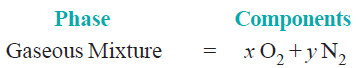– Hence a mixture of O2 and N2 has two components.

– In general the number of components of a gaseous mixture is given by the number of individual gases present.

### (3) Sodium chloride solution

– A solution of sodium chloride in water is a 1-phase system.

– Its composition (xNaCl.yH2O) can be expressed in terms of two chemical individuals, sodium chloride and water.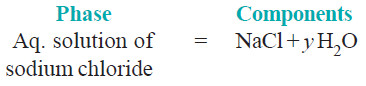– Therefore an aqueous solution of sodium chloride or any other solute is a two-component or
2-component system.

– A saturated solution of sodium chloride, in contact with excess solid sodium chloride has two
phase, namely aqueous solution and solid sodium chloride.

– The composition of both phase can be expressed in terms of two chemical individuals NaCl and H2O.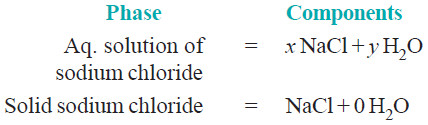– Hence a saturated solution of sodium chloride or any other solute in contact with solid solute,
is 2-component system.

### (4) Decomposition of Calcium carbonate.

– When calcium carbonate is heated in a closed vessel, the following equilibrium system results.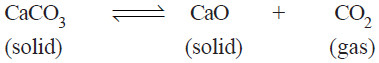– It has three phase : calcium carbonate, calcium oxide, and carbon dioxide.

– The composition of all the phase can be expressed in terms of any two of the three chemical substances in equilibrium.

– Let us select calcium oxide (CaO) and carbon dioxide (CO2) as the components. Then we can write,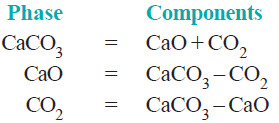– Again, selecting calcium carbonate (CaCO3) and calcium oxide (CaO) as the components, we have:– Thus decomposition of calcium carbonate is a 2-components system.

– Furthermore, as is clear from the above examples, by the components of a system is meant the number of chemical individuals and not any particular chemical substances by name.

### (5) Dissociation of Ammonium chloride.

– Ammonium chloride when heated in a closed vessel exists in equilibrium with the products of dissociation, ammonia (NH3) and hydrogen chloride gas (HCl).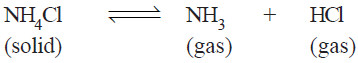– The system consists of two phase, namely, solid NH4Cl and the gaseous mixture containing NH3 and HCl.

– The constituents of the mixture are present in the same proportion in which they are combined in solid NH4Cl.

– The composition of both the phase can, therefore, be expressed in terms of the same chemical individual NH4Cl.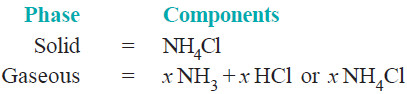– Thus dissociation of ammonium chloride is a one-component system.